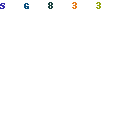Tiny Programmable Oscillator Spans Three Decades from 5Khz to 20Mhz

By merging a digital potentiometer with an oscillator chip, we can make a very small programmable oscillator (1 uMAX and 1 SOT23 package). Not only does this circuit take up very little board space but it can be controlled with only three signals from a microprocessor.

The MAX5160 is a 200K digital pot with 32 taps. To move up to the next tap, set UP/DOWN high and pulse INCREMENT low. To move down, set UP/DOWN low before pulsing INCREMENT.

The LTC1799 is a programmable oscillator from Linear Technology. The frequency of oscillation is proportional to the value of the set resistor between V+ and the SET pin. Internal to the chip is a divider which is controlled by the DIV pin. The following table illustrates the relationship between the divide ratio and the input on DIV:

 DIV Input Internal Divider Value (N) Ground 1 High Impedance 10 V+ 100

The equation for the oscillator frequency is:Where N is the divider value. A plot of this equation for each value of N and each of the tap locations on the digital potentiometer can be seen in the figure below. Note that there is some overlap between the curves for each value of N.In the schematic shown below, R1 was added to satisfy the minimum Rset requirement for the LTC1799. The circuit can be further simplified by fixing the DIV input and operating over a narrower output range.